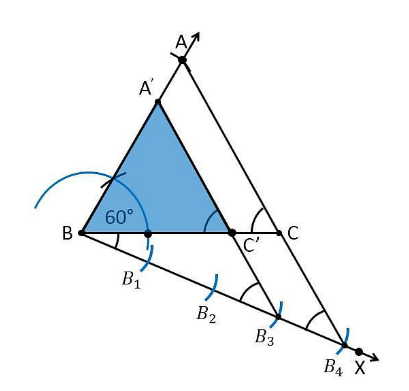# Draw a triangle ABC with side BC = 6 cm, AB = 5 cm and ∠ABC = 60°. Then construct a triangle whose sides are 3/4 of the corresponding sides of the triangle ABC. Give the justification of the construction

To construct a triangle from given conditions

1. Let us draw a ΔABC with base side BC = 6 cm, and AB = 5 cm and ∠ABC = 60°.

2. Draw a ray BX which forms an acute angle with BC on the opposite side of vertex A.

3. Position the 4 points (as 4 is greater in 3 and 4), such as B1, B2, B3, B4, on line segment BX.

4. Join the points B4C and also draw a line through B3, parallel to B4C intersecting the line segment BC at C’.

5. Draw a line through C’ parallel to the line AC which intersects the line AB at A’.

6. Therefore, ΔA’BC’ is the required triangle.Justification

Since the scale factor is 3/4, we need to prove

A’B = (3/4)AB

BC’ = (3/4)BC

A’C’= (3/4)AC

From the construction, we get A’C’ || AC

In ΔA’BC’ and ΔABC,

∴ ∠ A’C’B = ∠ACB (Corresponding angles)

∠B = ∠B (common)

∴ ΔA’BC’ ∼ ΔABC (From AA similarity criterion)

Since the corresponding sides of the similar triangle are in the same ratio, it becomes

Therefore, A’B/AB = BC’/BC= A’C’/AC

So, it becomes A’B/AB = BC’/BC= A’C’/AC = 3/4

Hence, justified.